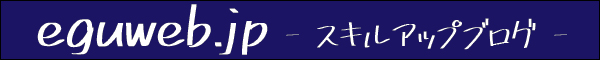# What is the AND function? Function to check if all conditions are TRUE / Google Sheets

## What is the AND function?

The AND function is a function that checks whether all conditions are TRUE .

## How to use the AND function

AND(logical_expression1, [logical_expression2, …])

Description　Returns TRUE if all arguments are logically TRUE. Returns FALSE if any argument is logically FALSE.

Logical Expression1A　formula or cell reference that contains a formula that represents a logical value (TRUE or FALSE), or an expression that can be coerced to a logical value.

Logical Expression 2　 – [Optional] Any other expression representing a repeatable logical value.

## Examples of using the AND function

(Example) For example, if the result of value A and value B is “both 5 or more”, you want to set it to TRUE.

=AND(A2>5,B2>5), the result can be determined.

For example, do the values ​​in the cells above and below match? can also be used for

Returns TRUE if the results in the cells above and below match.

## summary

You can use the AND function to determine if all conditions are met.

## What is the AND function?

The AND function is a function that checks whether all conditions are TRUE .

## How to use the AND function

AND(logical_expression1, [logical_expression2, …])

Description　Returns TRUE if all arguments are logically TRUE. Returns FALSE if any argument is logically FALSE.

Logical Expression1A　formula or cell reference that contains a formula that represents a logical value (TRUE or FALSE), or an expression that can be coerced to a logical value.

Logical Expression 2　 – [Optional] Any other expression representing a repeatable logical value.

## Examples of using the AND function

(Example) For example, if the result of value A and value B is “both 5 or more”, you want to set it to TRUE.

=AND(A2>5,B2>5), the result can be determined.

For example, do the values ​​in the cells above and below match? can also be used for

Returns TRUE if the results in the cells above and below match.

## summary

You can use the AND function to determine if all conditions are met.

## What is the AND function?

The AND function is a function that checks whether all conditions are TRUE .

## How to use the AND function

AND(logical_expression1, [logical_expression2, …])

Description　Returns TRUE if all arguments are logically TRUE. Returns FALSE if any argument is logically FALSE.

Logical Expression1A　formula or cell reference that contains a formula that represents a logical value (TRUE or FALSE), or an expression that can be coerced to a logical value.

Logical Expression 2　 – [Optional] Any other expression representing a repeatable logical value.

## Examples of using the AND function

(Example) For example, if the result of value A and value B is “both 5 or more”, you want to set it to TRUE.

=AND(A2>5,B2>5), the result can be determined.

For example, do the values ​​in the cells above and below match? can also be used for

Returns TRUE if the results in the cells above and below match.

## summary

You can use the AND function to determine if all conditions are met.